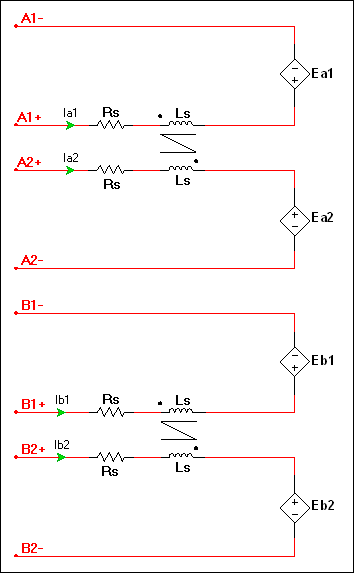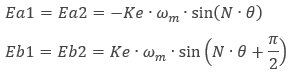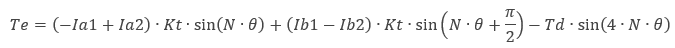OK

# Stepper 2 phase 2 winding model

Model assumptions

• Linear iron magnetization with no saturation
• Constant self and mutual inductance
• No interaction between phases
• Uniform air-gap
• No slot harmonics
• Perfect coupling between intra-phase windings

Configuration of the machine electrical systemwhere

Ke = speed constant
ωm = mechanical angular speed
θ = mechanical angle
= 90°/Step

Step = motor full size angle in degrees

The generated electromagnetic torque is:where

Kt = torque constant
Td = detent torque

Parameter Description
Armature inductance Armature winding inductance in henries. Ls as shown in the configuration of the machine’s electrical system.
Armature resistance Armature winding resistance in ohms. Rs as shown in the configuration of the machine’s electrical system.
Step angle Step angle in degrees. Used to calculate N.
Torque constant Torque constant in N•m/A. If neither this value nor Ke are specified on the datasheet, Kt can be estimated from any sample on the static holding torque (Th) and rated current curve.
Speed constant Speed constant in V•s/rad. This is typically equal to Kt. This is Ke in the Ea1 and Eb1 equations.
Detent torque Detent torque in N•m.
Shaft inertia J<sub>rotor</sub> on the machine model diagram in <a href="/help/components/machine-modeling/">Machine modeling</a>.
Shaft friction This is F<sub>rotor</sub> on the machine model diagram in <a href="/help/components/machine-modeling/">Machine modeling</a>.
Initial angular speed Rotational measurement of the shaft angle in rad/s at the start of the simulation.
Initial shaft angle Initial shaft angle in radians.